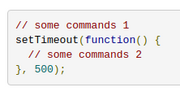# Differences between Arduino and Espruino code

Here are some common 'gotchas' you might encounter when moving from writing Arduino code to writing Espruino code. This might be especially helpful when 'porting' existing code.

## There is no `delay()` function

This is intentional, as adding a delay will stop Espruino doing other things. Instead, for large delays (>5ms) use `setTimeout` to execute the second set of code after a time period:

So the following:

``````// some commands 1
delay(500);
// some commands 2
``````

Becomes:

``````// some commands 1
setTimeout(function() {
// some commands 2
}, 500);
``````

## Time

Espruino doesn't have `millis()`. It has `getTime()`, which reports the current time in seconds as a floating point number.

Unlike most Arduinos, Espruino contains a Real Time Clock which allows it to keep track of time and date very accurately. This means you don't need an external RTC, and can instead use the Date class.

## There is no `loop()` function

A lot of Arduino code uses the following pattern:

``````void call_me_from_loop() {
if (millis() > last_time_called+1000) {
last_time_called = millis();

// do stuff
}
}
``````

In Espruino, this is really inefficient because it stops Espruino from sleeping when it knows it doesn't need to do anything. It's also needlessly complex.

Instead, use the following:

``````setInterval(function() {
// do stuff
}, 1000);
``````

## Analog IO

• `analogWrite` in Espruino takes a floating point value between 0 and 1, rather than an integer between 0 and 255
• `analogRead` in Espruino returns a floating point value between 0 and 1, rather than an integer between 0 and 1023
• Espruino is 3.3v (not 5v), so to get a voltage from `analogRead`, you have to multiply by 3.3 rather than 5. However we'd suggest multiplying by `E.getAnalogVRef()`, which measures the chip's voltage based on an internal voltage reference.
• If you want to read or write Audio, see Waveform - using `setInterval` directly probably won't work.

## Other IO

• `digitalRead` and `digitalWrite` can take an array of pins, in which case the value is treated as binary number
• There's no need to bit-bash SPI. You can create a software SPI instance on any pins using `new SPI()`

## Library Functions

• Strings can be added together, and numbers are converted to Strings automatically - so there's no need to have a separate `print` statement per value
• If you want to output a value to 2 decimal places, just use `console.log(value.toFixed(2))` - `console.log(x,2)` will just return the value of `x`, and then `2`

## Language

• Normal Arrays are sparse, so are not very efficient. You're best off using `Uint8Array` or similar.
• Code execution speed is not fantastic, see Performance. Instead of writing code, try and use library functions wherever possible.
• You can't write code that runs inside interrupts, and your code is never preempted. You can still measure pulses accurately though, as events from `setWatch` are timestampted to the nearest microsecond.

## Found something else?

Please let us know in the Forum...

This page is auto-generated from GitHub. If you see any mistakes or have suggestions, please let us know.